# Residents

Here are some of the guests staying at the inn..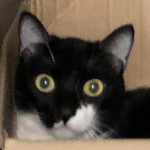Coco Here is the story of Coco.  Here is the story of Coco.  Here is the story of Coco.  Here is the story of Coco.  Here is the story of Coco.  Here is the story of Coco.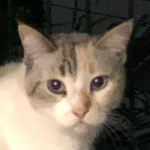Crispy Here is the story of Coco.  Here is the story of Coco.  Here is the story of Coco.  Here is the story of Coco.  Here is the story of Coco.  Here is the story of Coco.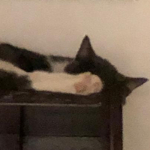Happy Here is the story of Coco.  Here is the story of Coco.  Here is the story of Coco.  Here is the story of Coco.  Here is the story of Coco.  Here is the story of Coco.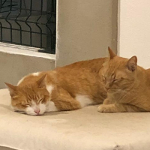Coco Here is the story of Coco.  Here is the story of Coco.  Here is the story of Coco.  Here is the story of Coco.  Here is the story of Coco.  Here is the story of Coco.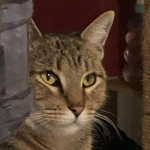Crispy Here is the story of Coco.  Here is the story of Coco.  Here is the story of Coco.  Here is the story of Coco.  Here is the story of Coco.  Here is the story of Coco.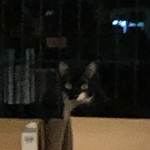Happy Here is the story of Coco.  Here is the story of Coco.  Here is the story of Coco.  Here is the story of Coco.  Here is the story of Coco.  Here is the story of Coco.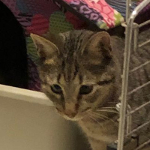Coco Here is the story of Coco.  Here is the story of Coco.  Here is the story of Coco.  Here is the story of Coco.  Here is the story of Coco.  Here is the story of Coco.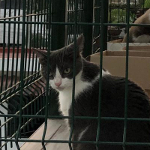Crispy Here is the story of Coco.  Here is the story of Coco.  Here is the story of Coco.  Here is the story of Coco.  Here is the story of Coco.  Here is the story of Coco.Happy Here is the story of Coco.  Here is the story of Coco.  Here is the story of Coco.  Here is the story of Coco.  Here is the story of Coco.  Here is the story of Coco.